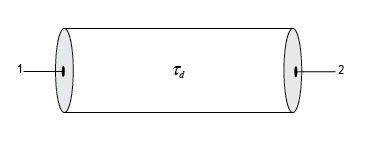# txlineDelayLossless

Create delay lossless transmission line

Since R2021a

## Description

Use the `txlineDelayLossless` object to create a delay lossless transmission line. You can also use the `txlineDelayLossless` object to model a delay lossless transmission line in an RF system using the `rfbudget` object or the RF Budget Analyzer app.## Creation

### Syntax

``dltxline = txlineDelayLossless``
``dltxline = txlineDelayLossless(Name,Value)``

### Description

````dltxline = txlineDelayLossless` creates a default delay lossless transmission line object.```

example

````dltxline = txlineDelayLossless(Name,Value)` sets Properties of the delay lossless transmission line using one or more name-value arguments. For example, ```dltxline = txlineDelayLossless('Z0',75)``` creates a delay lossless transmission line with an impedance of `75` ohms.```

## Properties

expand all

Name of the delay lossless transmission line, specified as a string scalar or character vector.

Example: `'Name','DLbased'`

Example: `dltxline.Name = 'DLbased'`

Characteristic impedance of the delay lossless transmission line, specified as a positive scalar in ohms.

Example: `'Z0',75`

Example: `dltxline.Z0 = 75`

Time delay (τd) in the delay lossless transmission line, specified as a positive scalar in seconds.

Example: `'TimeDelay',1e-9`

Example: `dltxline.TimeDelay = 1e-9`

Number of input and output ports of the delay lossless transmission line, returned as positive scalar.

Terminals of the delay lossless transmission line, returned as a cell array.

## Object Functions

 `sparameters` Calculate S-parameters for RF data, network, circuit, and matching network objects `groupdelay` Group delay of S-parameter object or RF filter object or RF Toolbox circuit object `noisefigure` Calculate noise figure of transmission lines, series RLC, and shunt RLC circuits

## Examples

collapse all

Create a delay lossless transmission line with a transmission delay of `5e-12` sec.

`dltxline = txlineDelayLossless('TimeDelay',5e-12);`

Calculate the group delay at `10` MHz.

`gd = groupdelay(dltxline,10e6)`
```gd = 5.0000e-12 ```

Calculate the noise figure at `10` MHz.

`nf = noisefigure(dltxline,10e6)`
```nf = 0 ```

## Version History

Introduced in R2021a